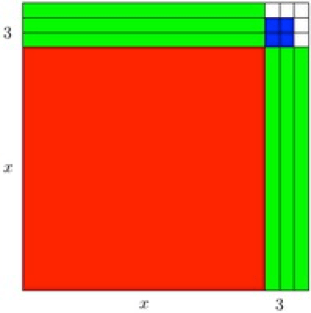# POLQ 2 | Lesson 2 | Explore (Completing the Square)# Completing the Square

Algebra tiles are useful for this lesson.  If you do not have algebra tiles to use, virtual algebra tiles can be found online.

Completing the square.

What expression is represented by the blue, green, and yellow algebra tiles below?

What would have to be added to make the diagram a complete square?So, the expression before completing the square was $$x^2+4x+2$$. After completing the square (adding 2), the new expression is $$(x^2+4x+4)-2$$ which when simplified is $$(x+2)^2-2$$.

Because two was added to the existing expression, to create a perfect square trinomial,  two also has to be subtracted to have an equivalent expression.

Use the same process for the the diagram below.